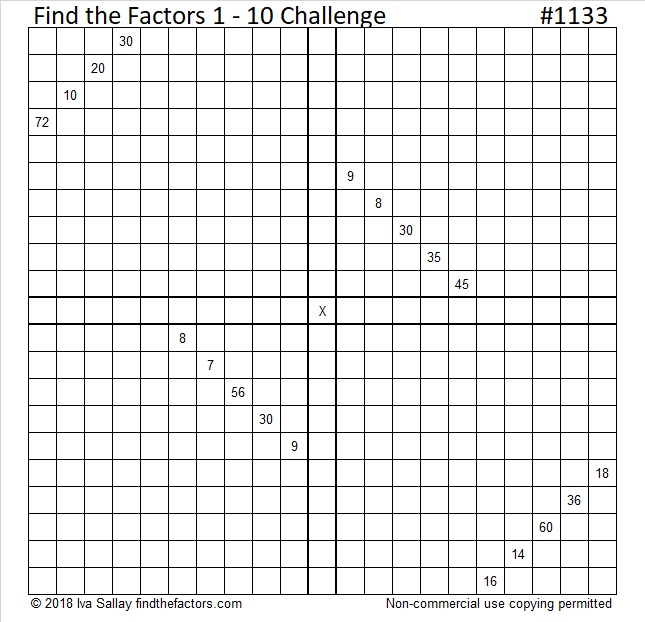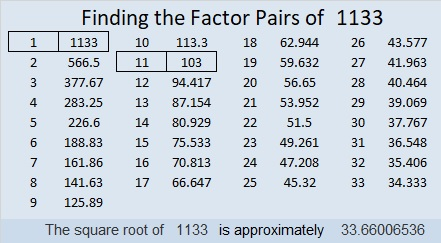# 1133 A Challenge Puzzle

I haven’t published a Find the Factors 1 – 10 Challenge puzzle for a while. Getting started on this one shouldn’t be difficult. The challenge will be in finishing it! Don’t guess and check. It can all be done using logic.Print the puzzles or type the solution in this excel file: 10-factors-1121-1133

Here are some facts about the number 1133:

Since both 11 and 33 are divisible by 11, we know 1133 can be evenly divided by 11.

• 1133 is a composite number.
• Prime factorization: 1133 = 11 × 103
• The exponents in the prime factorization are 1 and 1. Adding one to each and multiplying we get (1 + 1)(1 + 1) = 2 × 2 = 4. Therefore 1133 has exactly 4 factors.
• Factors of 1133: 1, 11, 103, 1133
• Factor pairs: 1133 = 1 × 1133 or 11 × 103
• 1133 has no square factors that allow its square root to be simplified. √1133 ≈ 33.660071133 is the sum of the twenty-one prime numbers from 13 to 101.
1133 is also the sum of the fifteen prime numbers from 43 to 107.

This site uses Akismet to reduce spam. Learn how your comment data is processed.